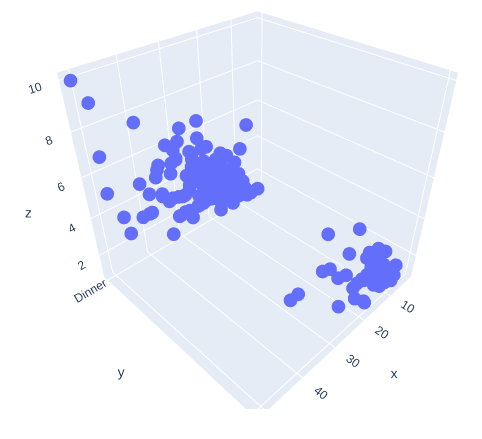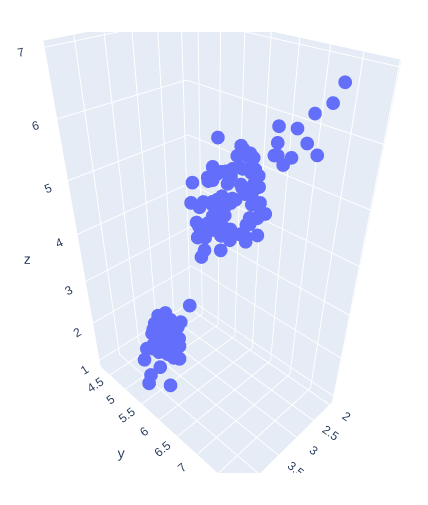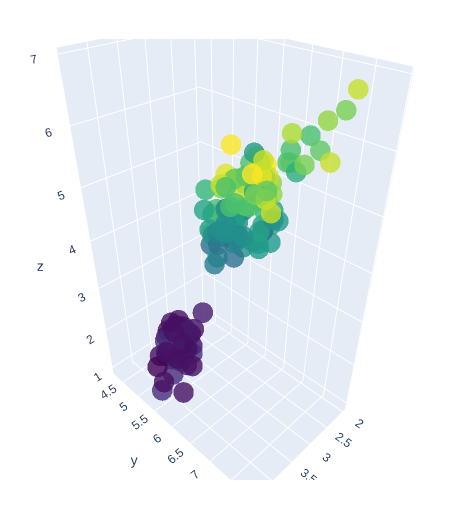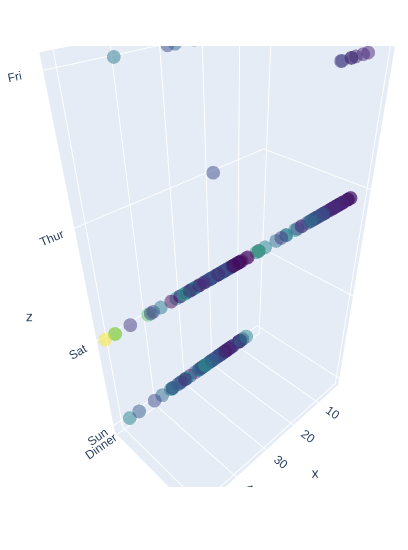# 3D Scatter Plot using graph_objects Class in Plotly-Python

Plotly is a Python library that is used to design graphs, especially interactive graphs. It can plot various graphs and charts like histogram, barplot, boxplot, spreadplot, and many more. It is mainly used in data analysis as well as financial analysis. plotly is an interactive visualization library.

## Scatter plot using graph_objects class

If a plotly express does not provide a good starting point, then it is possible to use go.Scatter3D class from plotly.graph_objects. Scatter plot are those charts in which data points are represented horizontally and on the vertical axis to show how one variable affect on another variable. The mode of the property decides the appearance of data points.

Syntax: plotly.graph_objects.Scatter3d(arg=None, connectgaps=None, customdata=None, customdatasrc=None, error_x=None, error_y=None, error_z=None, hoverinfo=None, hoverinfosrc=None, hoverlabel=None, hovertemplate=None, hovertemplatesrc=None, hovertext=None, hovertextsrc=None, ids=None, idssrc=None, legendgroup=None, line=None, marker=None, meta=None, metasrc=None, mode=None, name=None, opacity=None, projection=None, scene=None, showlegend=None, stream=None, surfaceaxis=None, surfacecolor=None, text=None, textfont=None, textposition=None, textpositionsrc=None, textsrc=None, texttemplate=None, texttemplatesrc=None, uid=None, uirevision=None, visible=None, x=None, xcalendar=None, xsrc=None, y=None, ycalendar=None, ysrc=None, z=None, zcalendar=None, zsrc=None, **kwargs)

Parameters:

x – Sets the x coordinates.

y – Sets the y coordinates.

z – Sets the z coordinates.

mode – Determines the drawing mode for this scatter trace.

Example 1:

## Python3

 `import` `plotly.express as px ` `import` `plotly.graph_objects as go ` ` `  `df ``=` `px.data.tips() ` ` `  `fig ``=` `go.Figure(data ``=``[go.Scatter3d(x ``=` `df[``'total_bill'``], ` `                                   ``y ``=` `df[``'time'``], ` `                                   ``z ``=` `df[``'tip'``], ` `                                   ``mode ``=``'markers'``)]) ` `fig.show()`

Output:Example 2:

## Python3

 `import` `plotly.express as px ` `import` `plotly.graph_objects as go ` ` `  `df ``=` `px.data.iris() ` ` `  `fig ``=` `go.Figure(data ``=``[go.Scatter3d(x ``=` `df[``'sepal_width'``], ` `                                   ``y ``=` `df[``'sepal_length'``], ` `                                   ``z ``=` `df[``'petal_length'``], ` `                                   ``mode ``=``'markers'``)]) ` `fig.show()`

Output:## Presenting 3D Scatter Plot with Color scaling and Marker Styling

In plotly, color scaling and marker styling are a way to represent the data more effectively, and it makes data more understandable.

Example 1:

## Python3

 `import` `plotly.express as px ` `import` `plotly.graph_objects as go ` ` `  `df ``=` `px.data.iris() ` ` `  `fig ``=` `go.Figure(data ``=``[go.Scatter3d(x ``=` `df[``'sepal_width'``], ` `                                   ``y ``=` `df[``'sepal_length'``], ` `                                   ``z ``=` `df[``'petal_length'``], ` `                                   ``mode ``=``'markers'``,  ` `                                   ``marker ``=` `dict``( ` `                                     ``size ``=` `12``, ` `                                     ``color ``=` `df[``'petal_width'``], ` `                                     ``colorscale ``=``'Viridis'``, ` `                                     ``opacity ``=` `0.8` `                                   ``) ` `)]) ` ` `  `fig.show()`

Output:Example 2:

## Python3

 `import` `plotly.express as px ` `import` `plotly.graph_objects as go ` ` `  `df ``=` `px.data.tips() ` ` `  `fig ``=` `go.Figure(data ``=``[go.Scatter3d(x ``=` `df[``'total_bill'``], ` `                                   ``y ``=` `df[``'time'``], ` `                                   ``z ``=` `df[``'day'``], ` `                                   ``mode ``=``'markers'``, ` `                                   ``marker ``=` `dict``( ` `                                     ``color ``=` `df[``'tip'``], ` `                                     ``colorscale ``=``'Viridis'``, ` `                                     ``opacity ``=` `0.5` `                                   ``) ` `)]) ` `fig.show()`

Output:Attention geek! Strengthen your foundations with the Python Programming Foundation Course and learn the basics.

To begin with, your interview preparations Enhance your Data Structures concepts with the Python DS Course.

My Personal Notes arrow_drop_upIf you like GeeksforGeeks and would like to contribute, you can also write an article using contribute.geeksforgeeks.org or mail your article to contribute@geeksforgeeks.org. See your article appearing on the GeeksforGeeks main page and help other Geeks.

Please Improve this article if you find anything incorrect by clicking on the "Improve Article" button below.

Article Tags :

Be the First to upvote.

Please write to us at contribute@geeksforgeeks.org to report any issue with the above content.